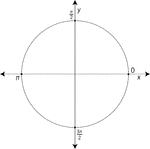### Unit Circle Labeled At Quadrantal Angles

Illustration of a unit circle (circle with a radius of 1) superimposed on the coordinate plane with…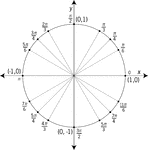### Unit Circle Labeled At Special Angles

Illustration of a unit circle (circle with a radius of 1) superimposed on the coordinate plane with…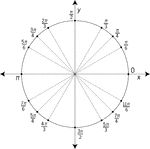### Unit Circle Labeled At Special Angles

Illustration of a unit circle (circle with a radius of 1) superimposed on the coordinate plane with…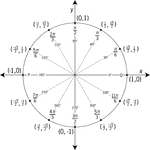### Unit Circle Labeled In 30° Increments With Values

Illustration of a unit circle (circle with a radius of 1) superimposed on the coordinate plane with…### Unit Circle Labeled In 45 ° Increments

Illustration of a unit circle (circle with a radius of 1) superimposed on the coordinate plane with…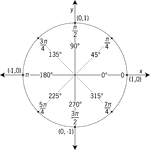### Unit Circle Labeled In 45° Increments With Values

Illustration of a unit circle (circle with a radius of 1) superimposed on the coordinate plane with…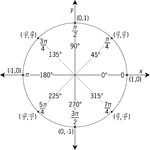### Unit Circle Labeled In 45° Increments With Values

Illustration of a unit circle (circle with a radius of 1) superimposed on the coordinate plane with…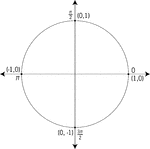### Unit Circle Labeled With Quadrantal Angles And Values

Illustration of a unit circle (circle with a radius of 1) superimposed on the coordinate plane. All…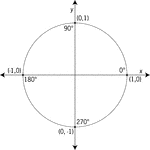### Unit Circle Labeled With Quadrantal Angles And Values

Illustration of a unit circle (circle with a radius of 1) superimposed on the coordinate plane with…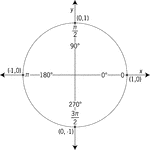### Unit Circle Labeled With Quadrantal Angles And Values

Illustration of a unit circle (circle with a radius of 1) superimposed on the coordinate plane with…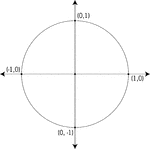### Unit Circle Labeled With Quadrantal Values

Illustration of a unit circle (circle with a radius of 1) superimposed on the coordinate plane. At each…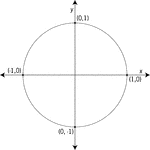### Unit Circle Labeled With Quadrantal Values

Illustration of a unit circle (circle with a radius of 1) superimposed on the coordinate plane with…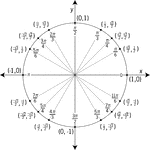### Unit Circle Labeled With Special Angles And Values

Illustration of a unit circle (circle with a radius of 1) superimposed on the coordinate plane with…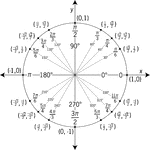### Unit Circle Labeled With Special Angles And Values

Illustration of a unit circle (circle with a radius of 1) superimposed on the coordinate plane with…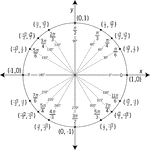### Unit Circle Labeled With Special Angles And Values

Illustration of a unit circle (circle with a radius of 1) superimposed on the coordinate plane with…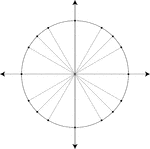### Unit Circle Marked At Special Angles

Illustration of a unit circle (circle with a radius of 1) superimposed on the coordinate plane. All…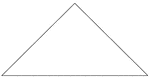### Isosceles Triangle degrees 90, 45, 45

An isosceles triangle with angles 90, 45, 45